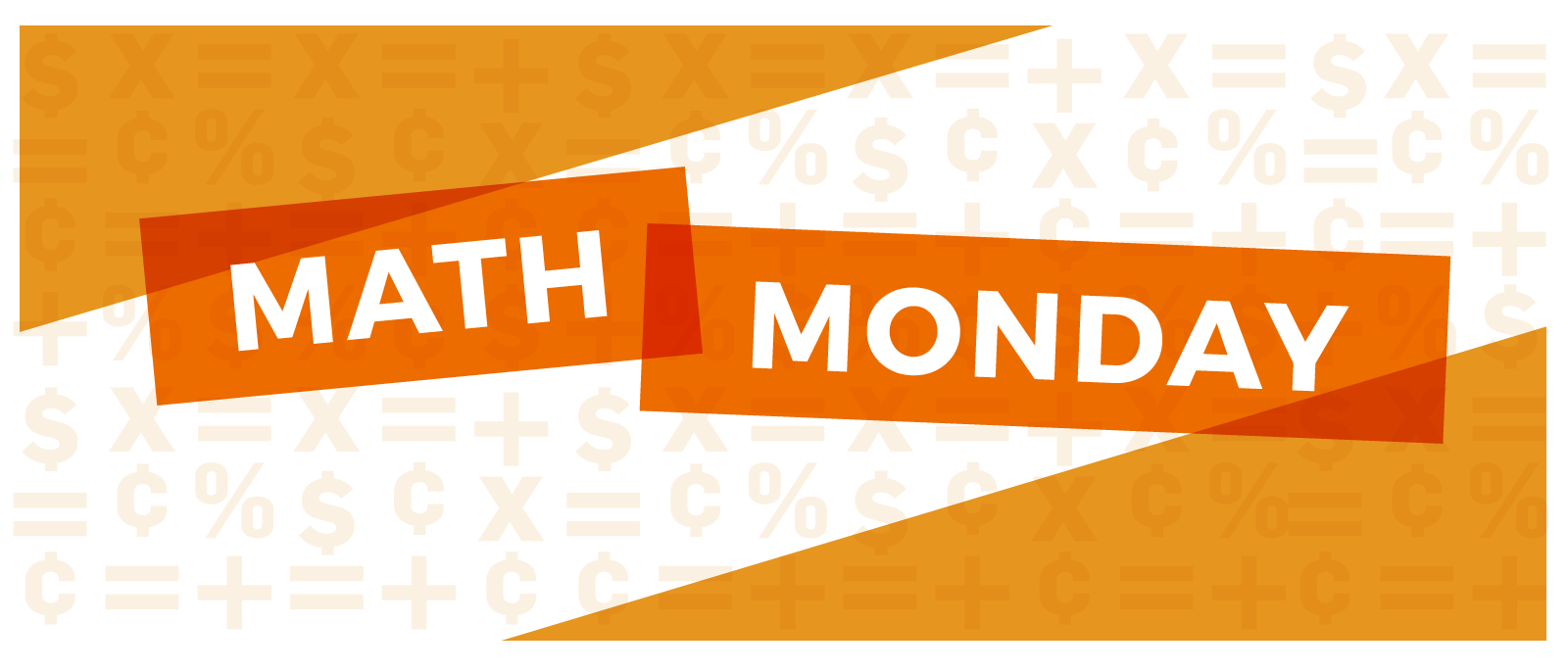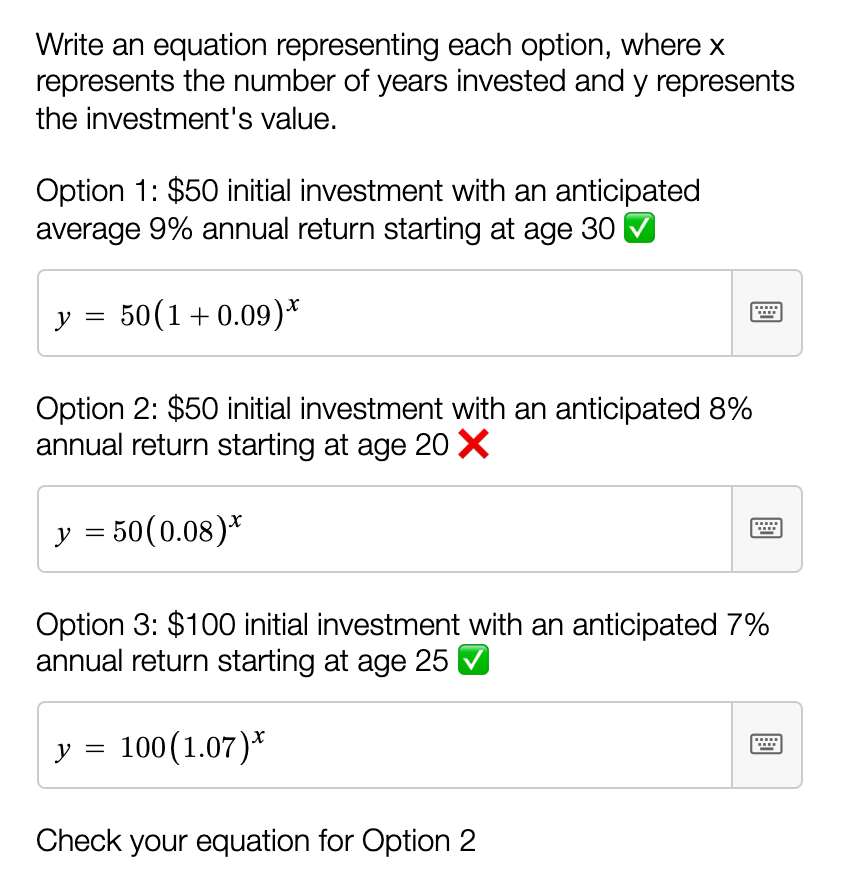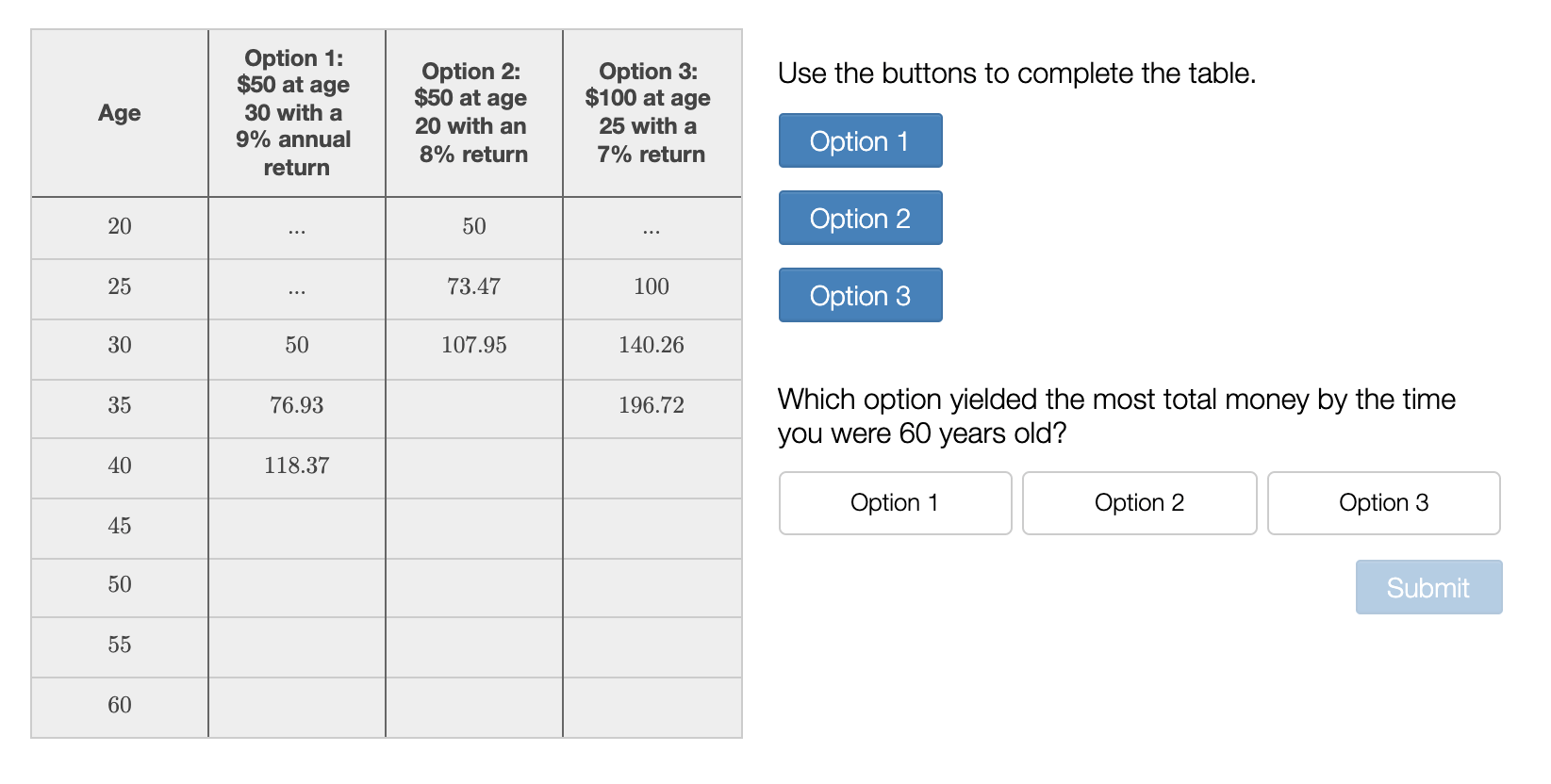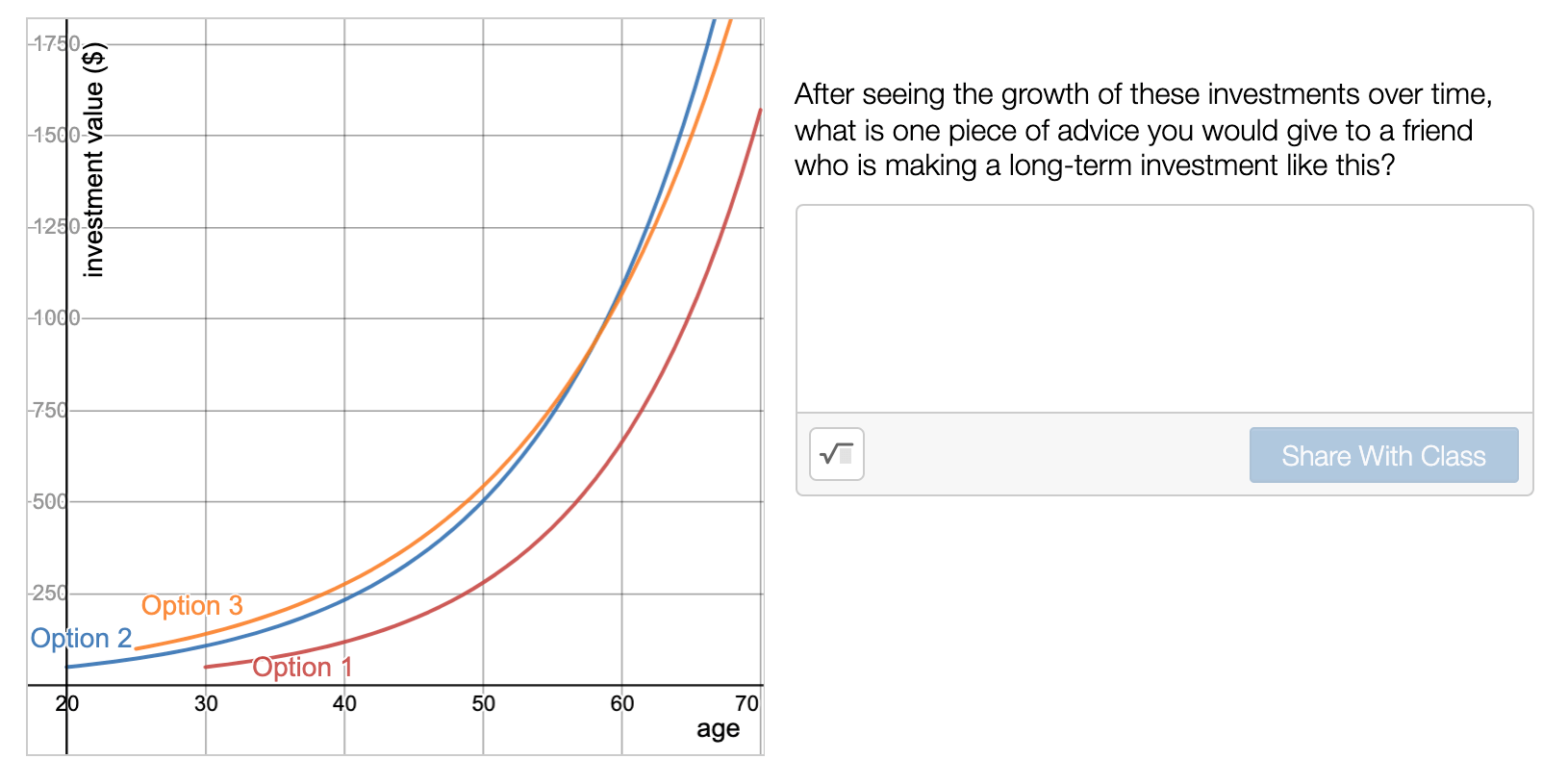Dec 12, 2021
Math

### MATH MONDAY: Investing and Exponential Change Desmos

What can exponential functions teach you about investing? In NGPF’s Desmos activity Investing and Exponential Change, students explore the impact of an investment's starting value, rate of return, and time invested. This activity is a part of the FA-5.5 Application in the new Intro to Investing & Exponential Functions unit.

The Set-Up

This activity is built around comparing three investment options:

• Option 1: Invest \$50 at age 30 with an anticipated 9% annual return
• Option 2: Invest \$50 at age 20 with an anticipated 8% annual return
• Option 3: Invest \$100 at age 25 with an anticipated 7% annual return

First, students make predictions: which account will be worth more after 10 years? 20 years? 30 years?Writing Equations and Calculating Investment Growth

Students then write equations for each option. This practices one of the lesson’s core skills: writing exponential equations in the form y = a(1±r)x. They can use the button to self-check their equations and you’ll receive a warning if students need to check more than three times.Students use their equation to complete the first three rows of the table to practice identifying the correct x-value and plugin it into an exponential equation. Then, they can use a button to auto-fill the remaining values.Reflection

Finally, students answer the reflection question “what is one piece of advice you would give to a friend who is making a long-term investment like this?” and read their classmates’ responses.Where to Find NGPF’s Desmos Activities

If you’re using our Financial Algebra curriculum, you’ll find Desmos activities built directly into the Lesson Guides and Application Answer Keys. To look through NGPF’s library of Desmos activities, you can check out our Financial Algebra Collection.

Want More?

Looking for an interesting question to kick off your lesson? Check out Question of the Day: How much would I need to save monthly to have \$1 million when I retire?